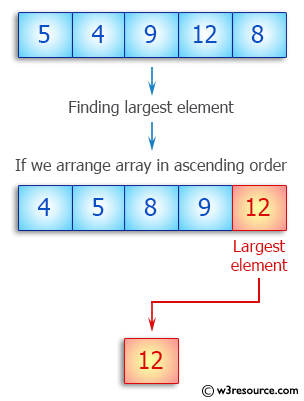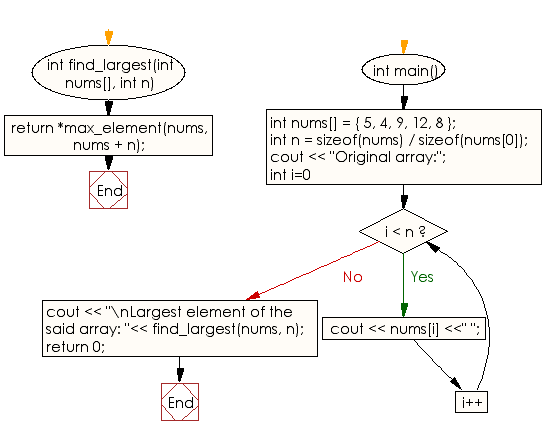﻿ C++ : Find the largest element of a given array of integers# C++ Exercises: Find the largest element of a given array of integers

## C++ Array: Exercise-1 with Solution

Write a C++ program to find the largest element of a given array of integers.

Pictorial Presentation:Sample Solution:

C++ Code :

``````#include<iostream>
using namespace std;
int find_largest(int nums[], int n) {
return *max_element(nums, nums + n);
}

int main() {
int nums[] = {
5,
4,
9,
12,
8
};
int n = sizeof(nums) / sizeof(nums);
cout << "Original array:";
for (int i=0; i < n; i++)
cout << nums[i] <<" ";

cout << "\nLargest element of the said array: "<< find_largest(nums, n);
return 0;
}
``````

Sample Output:

```Original array:5 4 9 12 8
Largest element of the said array: 12
```

Flowchart:C++ Code Editor:

What is the difficulty level of this exercise?

Test your Programming skills with w3resource's quiz.

﻿